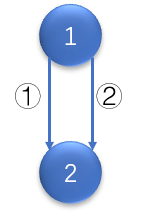# 洛谷 P2272/bzoj 1093 [ZJOI2007]最大半连通子图 题解【tarjan】【贪心】【树形DP】

一个比较难想的贪心+方案统计顺带树形DP。

6 6 20070603
1 2
2 1
1 3
2 4
5 6
6 4

3
3

## 题解：

对于最大半连通子图，如果满足任意两点之间都有关系，那么多画几个图，发现它不能有平行的边，也就是两点间路径唯一，可以是一棵有向树。不过对于任意两点，树就不满足这个性质，因此最大半连通子图有链的性质。同样可以发现，这里的环是可以被看作一个点的。环上的点能互相到达，因此环上的任一点都能通过环走到环上其他点的外向边去。因此，可以把一个大小为$k$的环缩为一个权值为$k$的点。这一点tarjan强连通分量缩点就可以完成。

接着求出最长链，这里可以用记忆化搜索+树形DP来实现。枚举每个没有被做过的点，进行DFS。每个点自身的方案数为1，如果它被多条最长路更新，那它的方案就是多条最长路方案之和。每次找到最长链更新答案，相同的最长链累加就可以了。

学会了tarjan缩点后对边的处理:在前向星中额外加入变量from，表示该边的起点。以from为第一关键字，n为第二关键字排序，然后unique去重。将ecnt更新到当前边数，重置head数组，把这些边按from和n重新相连即可。这个题要注意自环是有影响的，重边要消除。因为重边和自环虽然不会导致最长链持续更新下去，但是会更新方案数。根据子图的定义，它是由点集唯一确定的，因此下图中编号为1和2的边也只能算一种方案，因为点集${1,2}$被唯一确定。因此在记忆化搜索过程中记下方案数，每次更新时先改变答案，再更新答案有关的方案数就完成了。记得mod p。

## Code：

#include<cstdio>
#include<cstring>
#include<algorithm>
int min(int x,int y)
{
return x<y?x:y;
}
int max(int x,int y)
{
return x>y?x:y;
}
struct edge
{
int from,n,nxt;
edge(int from,int n,int nxt)
{
this->from=from;
this->n=n;
this->nxt=nxt;
}
edge()
{
nxt=-1;
}
friend bool operator <(edge a,edge b)
{
if(a.from!=b.from)
return a.from<b.from;
return a.n<b.n;
}
friend bool operator ==(edge a,edge b)
{
return a.from==b.from&&a.n==b.n;
}
}e;
{
}
int w;
int dfn,low,cnt=0;
int del,in,stk,top=0;
void tarjan(int x)
{
dfn[x]=++cnt;
low[x]=dfn[x];
in[x]=1;
stk[++top]=x;
{
if(dfn[e[i].n]&&!in[e[i].n])
continue;
if(dfn[e[i].n])
low[x]=min(low[x],dfn[e[i].n]);
else
{
tarjan(e[i].n);
low[x]=min(low[x],low[e[i].n]);
}
}
if(dfn[x]==low[x])
{
int cntt=0;
do
{
cntt++;
in[stk[top]]=0;
del[stk[top--]]=x;
}while(stk[top+1]!=x);
w[x]=cntt;
}

}
int p;
int used;
int ans=0,acnt=0;
int f;
void dfs(int x)
{
int tmp=0;
{
if(!used[e[i].n])
{
used[e[i].n]=1;
dfs(e[i].n);
}
if(ans==w[x]+w[e[i].n])
acnt+=f[e[i].n];
if(ans<w[x]+w[e[i].n])
{
ans=w[x]+w[e[i].n];
acnt=f[e[i].n];
}

if(tmp==w[e[i].n])
f[x]+=f[e[i].n];
if(tmp<w[e[i].n])
{
tmp=w[e[i].n];
f[x]=f[e[i].n];
}
}
if(ans==w[x])
acnt++;
if(ans<w[x])
{
ans=w[x];
acnt=1;
}
w[x]+=tmp;
f[x]%=p;
acnt%=p;
}
int main()
{
int n,m;
scanf("%d%d%d",&n,&m,&p);
int u,v;
for(int i=1;i<=m;i++)
{
scanf("%d%d",&u,&v);
}
for(int i=1;i<=n;i++)
if(!dfn[i])
tarjan(i);
for(int i=1;i<=n;i++)
{
e[j].n=del[e[j].n];
e[j].from=del[i];
}
std::sort(e,e+1+ecnt);
std::unique(e,e+1+ecnt);
for(int i=1;i<=ecnt;i++)
if(e[i].from<e[i-1].from||(e[i].from==e[i-1].from&&e[i].n<=e[i-1].n))
{
ecnt=i-1;
break;
}
for(int i=0;i<=ecnt;i++)
{
if(e[i].from==e[i].n)
continue;
}
for(int i=1;i<=n;i++)
f[i]=1;
for(int i=1;i<=n;i++)
if(del[i]==i&&!used[i])
{
used[del[i]]=1;
dfs(del[i]);
}
printf("%d\n",ans);
printf("%d\n",acnt%p);
return 0;
}

### 说点什么Subscribe

/* */